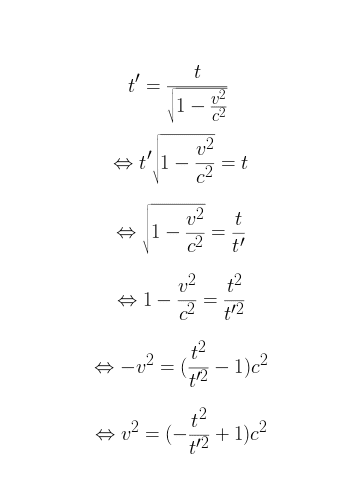# Express Velocity in Formula: Find Answer

• B
• hugo_faurand
Right, so if we take ## \frac{t^2}{t'^2} ## and express it as ##\left ( \frac{t}{t'} \right )^2##We get $$\beta = \sqrt{1- \left ( \frac{t}{t'} \right )^2}$$and $$\frac{t}{t'} = \sqrt{1- \beta^2}...$$In summary, the equation for expressing a velocity as a fraction of c is:$$v = c\sqrt{1-\frac{t^2}{t'^2}}$$f

#### hugo_faurand

Hello everyone.
This is not an homework but I'd like to express velocity ##v## in this formula :
$$t' = \frac{t}{\sqrt{1-\frac{v^2}{c^2}}}$$
I've found
$$v^2 = -(\frac{t^2}{t'^2}+1)c^2$$

I'm sure that is wrong but I don't manage to express ##v## and find the correct answer.

The 2nd equation looks to be close, with just one sign error. Can you show the steps you are trying to isolate v in the first equation?The 2nd equation looks to be close, with just one sign error. Can you show the steps you are trying to isolate v in the first equation?If it's a problem with a sign maybe this is correct :#### Attachments

If it's a problem with a sign maybe this is correct :
View attachment 227952
Yes, that looks fixed now, good work! What equation editor are you using BTW?Yes, that looks fixed now, good work! What equation editor are you using BTW?Yes, it works ! So finally we have :
$$v = \sqrt{(-\frac{t^2}{t'^2}+1)c^2}$$
Othewise I use ShareLaTex https://fr.sharelatex.com/ . Sorry, I'm french so it's the french version.

•berkeman
I'm french
Congrats on making the World Cup finals!Yes, it works ! So finally we have :
$$v = \sqrt{(-\frac{t^2}{t'^2}+1)c^2}$$
Othewise I use ShareLaTex https://fr.sharelatex.com/ . Sorry, I'm french so it's the french version.
Okay. So now you have c2 as a factor under the radical sign. Do you see a way to reduce this down further? And do you see a way of making the other part under the radical look cleaner?

•berkeman
Okay. So now you have c2 as a factor under the radical sign. Do you see a way to reduce this down further? And do you see a way of making the other part under the radical look cleaner?
In the radical we multiply by c^2 so we can just multiply the square root by c :
$$v = c\sqrt{(-\frac{t^2}{t'^2}+1)}$$
Also, dividing by a number is like multiplying by the inverse. So, I think we can have something like that :
$$v = c\sqrt{-{t^2}{t'^2}+1}$$

In the radical we multiply by c^2 so we can just multiply the square root by c :
Correct (although you'd normally phrase it as "take out a factor of c outside the square root", or something like that).
$$v = c^2\sqrt{(-\frac{t^2}{t'^2}+1)}$$
So this isn't right.
Also, dividing by a number is like multiply by the inverse. So, I think we can have something like that :
$$v = c^2\sqrt{-{t^2}{t'^2}+1}$$
Only if you define ##t'=1/t'##, which is a bit confusing. You could define something like ##f'=1/t'##, then use that. It's not a standard usage, though.

In the radical we multiply by c^2 so we can just multiply the square root by c :
$$v = c^2\sqrt{(-\frac{t^2}{t'^2}+1)}$$
Also, dividing by a number is like multiply by the inverse. So, I think we can have something like that :
$$v = c^2\sqrt{-{t^2}{t'^2}+1}$$

Ibex already pointed out that there is an error in your first equation.
For the second part, I was thinking more along the lines of what happens when you reverse the order of the terms.

Ibex already pointed out that there is an error in your first equation.
For the second part, I was thinking more along the lines of what happens when you reverse the order of the terms.

Reverse the order of the terms ? Maybe you mean put +1 before ## -\frac{t^2}{t'^2}##
So we have :
$$v = c\sqrt{1-\frac{t^2}{t'^2}}$$

Reverse the order of the terms ? Maybe you mean put +1 before ## -\frac{t^2}{t'^2}##
So we have :
$$v = c\sqrt{1-\frac{t^2}{t'^2}}$$
Right, Now consider that at times it is more convenient to work with velocities as expressed as a fraction of c such that ## \beta = \frac{v}{c} ##
so how would you express this equation using ##\beta ## ?

Right, Now consider that at times it is more convenient to work with velocities as expressed as a fraction of c such that ## \beta = \frac{v}{c} ##
so how would you express this equation using ##\beta ## ?
So we have :
$$\beta = \sqrt{1-\frac{t^2}{t'^2}}$$

So does the right hand side of the equation remind you of anything?

So does the right hand side of the equation remind you of anything?
It looks like what we have in the initial equation :
$$\sqrt{1-\frac{v^2}{c^2}}$$

But we change ##v## and ##c ## .

It looks like what we have in the initial equation :
$$\sqrt{1-\frac{v^2}{c^2}}$$

But we change ##v## and ##c ## .
Right, so if we take ## \frac{t^2}{t'^2} ## and express it as ##\left ( \frac{t}{t'} \right )^2##

We get
$$\beta = \sqrt{1- \left ( \frac{t}{t'} \right )^2}$$

and
$$\frac{t}{t'} = \sqrt{1- \beta^2}$$

•hugo_faurand